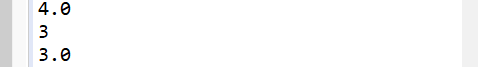• java中保留小数位数的方法 import java.math.BigDecimal; import java.text.DecimalFormat; import java.text.NumberFormat; 返回值是String类型 //方法一：最简便的方法，调用DecimalFormat（直接截掉不要的尾数，...
java中保留小数位数的方法
import java.math.BigDecimal;
import java.text.DecimalFormat;
import java.text.NumberFormat;
返回值是String类型
//方法一：最简便的方法，调用DecimalFormat（直接截掉不要的尾数，不四舍五入，返回值String类型）
 DecimalFormat df = new DecimalFormat(".00");
System.out.println(df.format(d));

//方法二：直接通过String类的format函数实现      （可以四舍五入，返回值String类型，%.2f表示保留后两位 ）
 System.out.println(String.format("%.2f", d));

//方法三：通过NumberFormat类实现，RoundingMode.HALF_DOWN表示 五舍六入，负数先取绝对值再五舍六入再负数，RoundingMode.HALF_UP:表示四舍五入，负数先取绝对值再五舍六入再负数。
  double d = 114.145
2 NumberFormat nf = NumberFormat.getNumberInstance();
3 // 保留两位小数
4 nf.setMaximumFractionDigits(2);
5 // 如果不需要四舍五入，可以使用RoundingMode.DOWN
6 nf.setRoundingMode(RoundingMode.UP);
7 System.out.println(nf.format(d));

返回值是double类型
Math.ceil()执行向上舍入，即它总是将数值向上舍入为最接近的整数；（数据类型还是double）
Math.floor()执行向下舍入，即它总是将数值向下舍入为最接近的整数；（数据类型还是double）
Math.round()执行标准舍入，即它总是将数值四舍五入为最接近的整数(数据类型变为int)。e是小数点后16位
System.out.println(Math.ceil(3.14159));
System.out.println(Math.round(3.14159));
System.out.println(Math.floor(3.14159));

结果是BigDecimal.ROUND_HALF_UP表示四舍五入，
BigDecimal.ROUND_HALF_DOWN表示五舍六入，
BigDecimal.ROUND_UP表示进位处理（就是直接加1），
BigDecimal.ROUND_DOWN表示直接去掉尾数。
  1 double d = 114.145;
2 d = (double) Math.round(d * 100) / 100;
3 System.out.println(d);

结果是
114.15
  1 double d = 114.145;
2 BigDecimal b = new BigDecimal(d);
3 d = b.setScale(2, BigDecimal.ROUND_HALF_UP).doubleValue();
4 System.out.println(d);

结果是
114.15
import java.math.BigDecimal; //引入这个包
public class Test {
public static void main(String[] args) {

double i = 3.856;

// 舍掉小数取整
System.out.println("舍掉小数取整:Math.floor(3.856)=" + (int) Math.floor(i));

// 四舍五入取整
System.out.println("四舍五入取整:(3.856)="
+ new BigDecimal(i).setScale(0, BigDecimal.ROUND_HALF_UP));

// 四舍五入保留两位小数
System.out.println("四舍五入取整:(3.856)="
+ new BigDecimal(i).setScale(2, BigDecimal.ROUND_HALF_UP));

// 凑整，取上限
System.out.println("凑整:Math.ceil(3.856)=" + (int) Math.ceil(i));

// 舍掉小数取整
System.out.println("舍掉小数取整:Math.floor(-3.856)=" + (int) Math.floor(-i));
// 四舍五入取整
System.out.println("四舍五入取整:(-3.856)="
+ new BigDecimal(-i).setScale(0, BigDecimal.ROUND_HALF_UP));

// 四舍五入保留两位小数
System.out.println("四舍五入取整:(-3.856)="
+ new BigDecimal(-i).setScale(2, BigDecimal.ROUND_HALF_UP));

// 凑整，取上限
System.out.println("凑整(-3.856)=" + (int) Math.ceil(-i));
}

}



展开全文• 注意：保留小数位数用用到BigDecimal类型做转换，所以要有这行引入jar包的程序： import java.math.BigDecimal; 1. 四舍五入保留两位小数： double a = 123.0232363869654697132695; a = new BigDecimal...
###注意：保留小数位数用用到BigDecimal类型做转换，所以要有这行引入jar包的程序：
import java.math.BigDecimal;

#1. 四舍五入保留两位小数：
double a = 123.0232363869654697132695;
a = new BigDecimal(a).setScale(2, BigDecimal.ROUND_HALF_UP).doubleValue();

#2. 各种保留小数点的方式：
只需设置setScale(int newScale, RoundingMode roundingMode)
的RoundingMode 参数即可：

RoundingMode
value

CEILING
向正无限大方向舍入的舍入模式。

DOWN
向零方向舍入的舍入模式。

FLOOR
向负无限大方向舍入的舍入模式。

HALF_DOWN
向最接近数字方向舍入的舍入模式，如果与两个相邻数字的距离相等，则向下舍入。

HALF_EVEN
向最接近数字方向舍入的舍入模式，如果与两个相邻数字的距离相等，则向相邻的偶数舍入。

HALF_UP
向最接近数字方向舍入的舍入模式，如果与两个相邻数字的距离相等，则向上舍入。 （就是四舍五入）

UNNECESSARY
用于断言请求的操作具有精确结果的舍入模式，因此不需要舍入。

UP
远离零方向舍入的舍入模式。

double doubleValue = -2.222;
String s = doubleValue+"";

//四舍五入     >=0.##5  进位     例如：  2.222 -> 2.22      2.225 -> 2.23                         -2.225 -> -2.23
doubleValue = new BigDecimal(s).setScale(2, BigDecimal.ROUND_HALF_UP).doubleValue();
System.out.println(doubleValue);

//     >0.##5  进位        例如： 2.222 -> 2.22        2.225 -> 2.22   2.226 -> 2.23       -2.225 -> -2.22
doubleValue = new BigDecimal(s).setScale(2, BigDecimal.ROUND_HALF_DOWN).doubleValue();
System.out.println(doubleValue);

//   正数进位，负数舍去 （趋向正无穷）              例如：  2.222 -> 2.23        2.225 -> 2.23                        -2.225 -> -2.22
doubleValue = new BigDecimal(s).setScale(2, BigDecimal.ROUND_CEILING).doubleValue();
System.out.println(doubleValue);

//   正数舍去，负数进位  （趋向负无穷）            例如：   2.222 -> 2.22        2.225 -> 2.22                         -2.225 -> -2.23
doubleValue = new BigDecimal(s).setScale(2, BigDecimal.ROUND_FLOOR).doubleValue();
System.out.println(doubleValue);

//直接进位（远离0）     例如：2.222 -> 2.23       2.225 -> 2.23                             -2.225 -> -2.23
doubleValue = new BigDecimal(s).setScale(2, BigDecimal.ROUND_UP).doubleValue();
System.out.println(doubleValue);

//直接舍去（趋向0）     例如：2.222 -> 2.23       2.225 -> 2.22                             -2.225 -> -2.22
doubleValue = new BigDecimal(s).setScale(2, BigDecimal.ROUND_DOWN).doubleValue();
System.out.println(doubleValue);




展开全文• 本人正在自学Java，有些编程题要求输出小数保留n位小数，想请问各位大神们，在Java中有哪些可以实现以固定精度输出小数的方法？方法最好给多一些并且给出示例代码，谢谢。
• java.text.DecimalFormat df=new java.text.DecimalFormat("#.##");  double d=3.14159;  System.out.println(df.format(d)); 第二种： java.math.BigDecimal  BigDecimal bd = new BigDecimal("3....
第一种：java.text.DecimalFormat df=new java.text.DecimalFormat("#.##"); double d=3.14159; System.out.println(df.format(d));第二种：java.math.BigDecimal BigDecimal bd = new BigDecimal("3.14159265"); bd = bd.setScale(2,BigDecimal.ROUND_HALF_UP);第三种：long l = Math.round(3.14159*100); //四舍五入 double ret = l/100.0; //注意：使用 100.0 而不是 100 第四种：double d = 13.4324; d=((int)(d*100))/100;
展开全文小数位数
• 今天项目需要更改时长的显示方式，规定必须保留两位小数，刚才看简书的时候正好看到一个指定保留小数位数的工具类的文章，在此基础上，做了一点小修改，用起来更加方便了，有需要的朋友尽管撸走 DecimalUtils 类：...

今天项目中需要更改时长的显示方式，规定必须保留两位小数，刚才看简书的时候正好看到一个指定保留小数位数的工具类的文章，在此基础上，做了一点小修改，用起来更加方便了，有需要的朋友尽管撸走

DecimalUtils 类：

import java.math.BigDecimal;
import java.math.RoundingMode;
import java.text.DecimalFormat;

/**
* Created by Sean on 17/3/10.
*/

public class DecimalUtils {

/**
* 按四舍五入保留指定小数位数，位数不够用0补充
* @param o 格式化前的小数
* @param newScale 保留小数位数
* @return 格式化后的小数
*/
public static String formatDecimalWithZero(Object o, int newScale) {
return String.format("%." + newScale + "f", o);
}

/**
* 按四舍五入保留指定小数位数，位数不够用0补充
* @param d 格式化前的小数
* @param newScale 保留小数位数
* @return 格式化后的小数
*/
public static String formatDecimalWithZero(double d, int newScale) {
String pattern = "0.";
for (int i = 0; i < newScale; i++) {
pattern += "0";
}
DecimalFormat df = new DecimalFormat(pattern);
return df.format(d);
}

/**
* 按四舍五入保留指定小数位数，位数不够用0补充
* @param d 格式化前的小数 String形式
* @param newScale 保留小数位数
* @return 格式化后的小数
*/
public static String formatDecimalWithZero(String d, int newScale) {
String pattern = "0.";
for (int i = 0; i < newScale; i++) {
pattern += "0";
}
DecimalFormat df = new DecimalFormat(pattern);
return df.format(Double.valueOf(d));
}

/**
* 按四舍五入保留指定小数位数，小数点后仅保留有效位数
* @param d 格式化前的小数
* @param newScale 保留小数位数
* @return 格式化后的小数
*/
public static String formatDecimal(double d, int newScale) {
String pattern = "#.";
for (int i = 0; i < newScale; i++) {
pattern += "#";
}
DecimalFormat df = new DecimalFormat(pattern);
return df.format(d);
}

/**
* 按四舍五入保留指定小数位数，小数点后仅保留有效位数
* @param d 格式化前的小数
* @param newScale 保留小数位数
* @return 格式化后的小数
*/
public static String formatDecimal(String d, int newScale) {
String pattern = "#.";
for (int i = 0; i < newScale; i++) {
pattern += "#";
}
DecimalFormat df = new DecimalFormat(pattern);
return df.format(Double.valueOf(d));
}

/**
* 按指定舍入模式保留指定小数位数
* @param d 格式化前的小数
* @param newScale 保留小数位数
* @param roundingMode 舍入模式
*        (RoundingMode.UP始终进一/DOWN直接舍弃/
*         CEILING正进负舍/FLOOR正舍负进/
*         HALF_UP四舍五入/HALF_DOWN五舍六进/
*         HALF_EVEN银行家舍入法/UNNECESSARY抛出异常)
* @return 格式化后的小数
*/
public static double formatDecimal(double d, int newScale, RoundingMode roundingMode) {
BigDecimal bd = new BigDecimal(d).setScale(newScale, roundingMode);
return bd.doubleValue();
}

/**
* 按指定舍入模式保留指定小数位数
* @param d 格式化前的小数
* @param newScale 保留小数位数
* @param roundingMode 舍入模式
*        (RoundingMode.UP始终进一/DOWN直接舍弃/
*         CEILING正进负舍/FLOOR正舍负进/
*         HALF_UP四舍五入/HALF_DOWN五舍六进/
*         HALF_EVEN银行家舍入法/UNNECESSARY抛出异常)
* @return 格式化后的小数
*/
public static double formatDecimal(String d, int newScale, RoundingMode roundingMode) {
BigDecimal bd = new BigDecimal(Double.valueOf(d)).setScale(newScale, roundingMode);
return bd.doubleValue();
}

}

原文地址
展开全文• 今天项目需要更改时长的显示方式，规定必须保留两位小数，刚才看简书的时候正好看到一个指定保留小数位数的工具类的文章，在此基础上，做了一点小修改，用起来更加方便了，有需要的朋友尽管撸走DecimalUtils 类：...
• 方式一：四舍五入double f = 111231.5585;BigDecimal b = new BigDecimal(f);double f1 = b.setScale(2, BigDecimal.ROUND_HALF_UP).doubleValue();保留两位小数------------------------------------------...
• java保留两位小数问题：方式一：四舍五入double f = 111231.5585;BigDecimal b = new BigDecimal(f);double f1 = b.setScale(2, BigDecimal.ROUND_HALF_UP).doubleValue();保留两位小数----------------------------...
• 方式一：四舍五入double f = 111231.5585;BigDecimal b = new BigDecimal(f);double f1 = b.setScale(2, BigDecimal.ROUND_HALF_UP).doubleValue();保留两位小数方式二：java.text.DecimalFormat df =new ...
• java保留两位小数问题： 方式一： 四舍五入 double f = 111231.5585; BigDecimal b = new BigDecimal(f); double f1 = b.setScale(2, BigDecimal.ROUND_HALF_UP).doubleValue(); 方式二： java.text....
• 环境jdk：1.7前言之前，我曾...问题的主要因为是我没有弄清保留有效位和保留小数位是互斥的关系在此之前，我想写出一个通用的方法，就是既能保留有效位，又能保留小数位；/** * 相乘 保留是有效数字，而非有效的小数...
• decimal保留指定位数小数的时候，.NET自带的方法都是四舍五入的。项目遇到分摊金额的情况，最后一条的金额=总金额-已经分摊金额的和。format保留两位小数这样可能导致最后一条分摊的时候是负数，所以自己写了一......
• 当时的我，没有弄清楚有效位和小数位数其实是互斥的！ 导致在之后的工作业务出现了问题； 今天特意记录下； 问题的主要因为是我没有弄清保留有效位和保留小数位是互斥的关系 在此之前，我想写出一个通用的方法...
• 方式一： 四舍五入 double f = 111231.5585; BigDecimal b = new BigDecimal(f); double f1 = b.setScale(2, BigDecimal.ROUND_HALF_UP).doubleValue();...保留两位小数 方式二： java.text.DecimalFo...
• java中保留小数的常见方式 public class Test { public static void main(String[] args) { double a = 3.14159265357; double b = 3.14159265357; double c = 3.14159265357; // todo： 方法一 // ...
• java中保留任意位数的小数/java中四舍五入/获得任意位数的方法 /java中double类型保留小数位数 、 float保留小数位数
• java中保留小数示例：/*** 1.BigDecimal常用于金额的计算，* 超过16位使用*/public void m1() {BigDecimal bg = new BigDecimal(f);double f1 = bg.setScale(2, BigDecimal.ROUND_HALF_UP).doubl...
• 本人曾经在2018年毕业时的山东浪潮集团笔试题遇见了这种要求最终结果保留两位小数的情况，对于那些要参加笔试或者CCF考试的兄弟一定要注意审题；import java.math.BigDecimal; import java.text.DecimalFormat; ...
• import java.math.BigDecimal; /* * 计算并输出1/3.0的值，保留小数点后2位 * 方式一：四舍五入 */ public class Main{ public static void main(String[] args){ double n = 1/3.0; BigDecimal b = new ...
• title: java中小数点后保留指定小数位数方法 date: 2019-07-01 14:53:42 tags: java 本文介绍java中几种保留小数点后指定小位数的方法,下面以保留两位为例 package study; import java.math.BigDecimal; import ......

# java中保留小数位数java 订阅